Updating search results...

# 2740 Results

View
Selected filters:
• Mathematics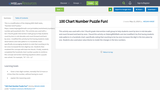Unrestricted Use
CC BY
Rating
0.0 stars

This is a modification of the Helping With Math tasks, "Number Grid Puzzles," https://www.helpingwithmath.com/printables/worksheets/numbers/1nbt4-number-grid-puzzles01.htm. This activity was used with a tier 3 fourth grade intervention math group to help students count by tens in mid-decades and count forward and back by ones. I modified this activity by first having students make observations and note patterns in a hundreds chart; specifically encouraging students to note that counting on by ten ones increased the tens digit by one. Students then modeled this concept with base-ten blocks. Finally, students completed the hundreds chart number puzzles to reinforce this concept and wrote matching equations when a puzzle was solved. For example, "47 = 35 + 12."

Subject:
Mathematics
Material Type:
Author:
MEGAN HINDE
08/02/2019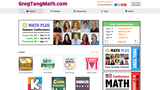Restricted Use
Rating
0.0 stars

This is a game where students practice adding and decomposing sums up to 12.  The game is for 2 players and requires two six-sided dice, and a 10-Pin Limbo! game sheet.

Subject:
Mathematics
Material Type:
Game
Provider:
Creative Smarts, Inc.
01/18/2017Conditional Remix & Share Permitted
CC BY-NC-SA
Rating
0.0 stars

This is a task from the Illustrative Mathematics website that is one part of a complete illustration of the standard to which it is aligned. Each task has at least one solution and some commentary that addresses important asects of the task and its potential use. Here are the first few lines of the commentary for this task: First pose the question: Here are four triangles. What do all of these triangles have in common? What makes them different from the figures that are no...

Subject:
Mathematics
Material Type:
Activity/Lab
Provider:
Illustrative Mathematics
Provider Set:
Illustrative Mathematics
Author:
Illustrative Mathematics
05/24/2013Conditional Remix & Share Permitted
CC BY-NC-SA
Rating
0.0 stars

This is a task from the Illustrative Mathematics website that is one part of a complete illustration of the standard to which it is aligned. Each task has at least one solution and some commentary that addresses important asects of the task and its potential use. Here are the first few lines of the commentary for this task: Materials * A copy of Grandfather Tang's Story by Ann Tompert * One set of tangrams for each student (see note in commentary) * A set of tangrams for t...

Subject:
Mathematics
Material Type:
Activity/Lab
Provider:
Illustrative Mathematics
Provider Set:
Illustrative Mathematics
Author:
Illustrative Mathematics
05/29/2013Conditional Remix & Share Permitted
CC BY-NC-SA
Rating
0.0 stars

This is a task from the Illustrative Mathematics website that is one part of a complete illustration of the standard to which it is aligned. Each task has at least one solution and some commentary that addresses important asects of the task and its potential use. Here are the first few lines of the commentary for this task: Materials - 2 clear plastic cups for each pair of students - 4 bean seeds for each pair - soil - unifix cubes - a plant or math journal to record data ...

Subject:
Mathematics
Material Type:
Activity/Lab
Provider:
Illustrative Mathematics
Provider Set:
Illustrative Mathematics
Author:
Illustrative Mathematics
04/17/2013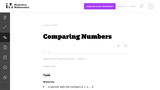Conditional Remix & Share Permitted
CC BY-NC-SA
Rating
0.0 stars

This is a task from the Illustrative Mathematics website that is one part of a complete illustration of the standard to which it is aligned. Each task has at least one solution and some commentary that addresses important asects of the task and its potential use. Here are the first few lines of the commentary for this task: Materials A spinner with the numbers 0, 1, 2, ... 9 A spinner with the decades 00, 10, 20, ... 90 Math journal or teacher-made worksheet Pencil Actions...

Subject:
Mathematics
Material Type:
Activity/Lab
Provider:
Illustrative Mathematics
Provider Set:
Illustrative Mathematics
Author:
Illustrative Mathematics
04/19/2013Conditional Remix & Share Permitted
CC BY-NC-SA
Rating
0.0 stars

This is a task from the Illustrative Mathematics website that is one part of a complete illustration of the standard to which it is aligned. Each task has at least one solution and some commentary that addresses important aspects of the task and its potential use.

Subject:
Numbers and Operations
Material Type:
Activity/Lab
Provider:
Illustrative Mathematics
Provider Set:
Illustrative Mathematics
10/10/2017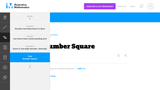Conditional Remix & Share Permitted
CC BY-NC-SA
Rating
0.0 stars

This is a task from the Illustrative Mathematics website that is one part of a complete illustration of the standard to which it is aligned. Each task has at least one solution and some commentary that addresses important aspects of the task and its potential use.

Subject:
Numbers and Operations
Material Type:
Activity/Lab
Provider:
Illustrative Mathematics
Provider Set:
Illustrative Mathematics
10/10/2017Conditional Remix & Share Permitted
CC BY-NC-SA
Rating
0.0 stars

This is a task from the Illustrative Mathematics website that is one part of a complete illustration of the standard to which it is aligned. Each task has at least one solution and some commentary that addresses important asects of the task and its potential use. Here are the first few lines of the commentary for this task: Materials For each pair: * 2 ten-sided dice with the numbers 0 to 9 or two spinners with the numbers 0 to 9 * Base-10 blocks, linking cubes, or bundled...

Subject:
Mathematics
Material Type:
Activity/Lab
Provider:
Illustrative Mathematics
Provider Set:
Illustrative Mathematics
Author:
Illustrative Mathematics
05/24/2013Conditional Remix & Share Permitted
CC BY-NC-SA
Rating
0.0 stars

This is a task from the Illustrative Mathematics website that is one part of a complete illustration of the standard to which it is aligned. Each task has at least one solution and some commentary that addresses important asects of the task and its potential use. Here are the first few lines of the commentary for this task: Materials Set of cards from 1-100, these can be purchased or created using a black marker and index cards Setup Sort the 1-100 cards into sequential gr...

Subject:
Mathematics
Material Type:
Activity/Lab
Provider:
Illustrative Mathematics
Provider Set:
Illustrative Mathematics
Author:
Illustrative Mathematics
03/16/2013Conditional Remix & Share Permitted
CC BY-NC-SA
Rating
0.0 stars

This is a task from the Illustrative Mathematics website that is one part of a complete illustration of the standard to which it is aligned. Each task has at least one solution and some commentary that addresses important asects of the task and its potential use. Here are the first few lines of the commentary for this task: Materials 20 counters or linking cubes per pair of students pencil copy of the problem Actions The teacher poses the problem: Bo bought 20 tickets to p...

Subject:
Mathematics
Material Type:
Activity/Lab
Provider:
Illustrative Mathematics
Provider Set:
Illustrative Mathematics
Author:
Illustrative Mathematics
05/05/2013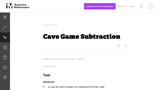Conditional Remix & Share Permitted
CC BY-NC-SA
Rating
0.0 stars

This is a task from the Illustrative Mathematics website that is one part of a complete illustration of the standard to which it is aligned. Each task has at least one solution and some commentary that addresses important asects of the task and its potential use. Here are the first few lines of the commentary for this task: Materials * A cup for each student to represent his/her cave * Counters * Recording sheet Actions The teacher begins by counting out a certain number o...

Subject:
Mathematics
Material Type:
Activity/Lab
Provider:
Illustrative Mathematics
Provider Set:
Illustrative Mathematics
Author:
Illustrative Mathematics
03/25/2014Conditional Remix & Share Permitted
CC BY-NC-SA
Rating
0.0 stars

This is a task from the Illustrative Mathematics website that is one part of a complete illustration of the standard to which it is aligned. Each task has at least one solution and some commentary that addresses important asects of the task and its potential use. Here are the first few lines of the commentary for this task: Materials A large set of dominoes to affix to a whiteboard or place in a pocket chart, or a regular set to use on a document projector. One set of domi...

Subject:
Mathematics
Material Type:
Activity/Lab
Provider:
Illustrative Mathematics
Provider Set:
Illustrative Mathematics
Author:
Illustrative Mathematics
05/24/2013Conditional Remix & Share Permitted
CC BY-NC-SA
Rating
0.0 stars

This is a task from the Illustrative Mathematics website that is one part of a complete illustration of the standard to which it is aligned. Each task has at least one solution and some commentary that addresses important aspects of the task and its potential use.

Subject:
Algebra
Material Type:
Activity/Lab
Provider:
Illustrative Mathematics
Provider Set:
Illustrative Mathematics
10/10/2017Conditional Remix & Share Permitted
CC BY-NC-SA
Rating
0.0 stars

This is a task from the Illustrative Mathematics website that is one part of a complete illustration of the standard to which it is aligned. Each task has at least one solution and some commentary that addresses important asects of the task and its potential use. Here are the first few lines of the commentary for this task: Materials * Fact Family work sheets * Blank addition and subtraction frames (2 of each) * Numbers cards in fact-family sets Actions As a whole class, t...

Subject:
Mathematics
Material Type:
Activity/Lab
Provider:
Illustrative Mathematics
Provider Set:
Illustrative Mathematics
Author:
Illustrative Mathematics
09/06/2013Conditional Remix & Share Permitted
CC BY-NC-SA
Rating
0.0 stars

This is a task from the Illustrative Mathematics website that is one part of a complete illustration of the standard to which it is aligned. Each task has at least one solution and some commentary that addresses important asects of the task and its potential use. Here are the first few lines of the commentary for this task: Write as many equations for each picture as you can. Use the numbers 4, 1, and 5. Here are some equations for this picture. 4+1=5 \hskip4em 5 = 4+1 5-1...

Subject:
Mathematics
Material Type:
Activity/Lab
Provider:
Illustrative Mathematics
Provider Set:
Illustrative Mathematics
Author:
Illustrative Mathematics
03/25/2014Conditional Remix & Share Permitted
CC BY-NC-SA
Rating
0.0 stars

This is a task from the Illustrative Mathematics website that is one part of a complete illustration of the standard to which it is aligned. Each task has at least one solution and some commentary that addresses important asects of the task and its potential use. Here are the first few lines of the commentary for this task: Materials * Link-cubes or snap-cubes (2 colors for each student or pair of students) * A die * Paper and pencil Actions 1. Roll the die. 2. Using a sin...

Subject:
Mathematics
Material Type:
Activity/Lab
Provider:
Illustrative Mathematics
Provider Set:
Illustrative Mathematics
Author:
Illustrative Mathematics
09/18/2013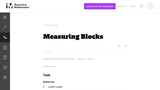Conditional Remix & Share Permitted
CC BY-NC-SA
Rating
0.0 stars

This is a task from the Illustrative Mathematics website that is one part of a complete illustration of the standard to which it is aligned. Each task has at least one solution and some commentary that addresses important asects of the task and its potential use. Here are the first few lines of the commentary for this task: Materials * Unifix cubes * Large blocks in different sizes or varying lengths of sentence strips Note: The large blocks or the cut-up lengths of senten...

Subject:
Mathematics
Material Type:
Activity/Lab
Provider:
Illustrative Mathematics
Provider Set:
Illustrative Mathematics
Author:
Illustrative Mathematics
03/18/2014Conditional Remix & Share Permitted
CC BY-NC-SA
Rating
0.0 stars

This is a task from the Illustrative Mathematics website that is one part of a complete illustration of the standard to which it is aligned. Each task has at least one solution and some commentary that addresses important asects of the task and its potential use. Here are the first few lines of the commentary for this task: Materials The Very Hungry Caterpillar by Eric Carle The students work individually or in pairs. Each student or pair needs: Three ten-frames for each s...

Subject:
Mathematics
Material Type:
Activity/Lab
Provider:
Illustrative Mathematics
Provider Set:
Illustrative Mathematics
Author:
Illustrative Mathematics
04/24/2013Conditional Remix & Share Permitted
CC BY-NC-SA
Rating
0.0 stars

This problem helps students practice adding three numbers whose sum are 20 or less.

Subject:
Mathematics
Numbers and Operations
Material Type:
Activity/Lab
Provider:
Illustrative Mathematics
Provider Set:
Illustrative Mathematics
Author:
Illustrative Mathematics
09/10/2012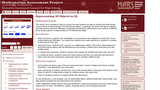Only Sharing Permitted
CC BY-NC-ND
Rating
0.0 stars

This lesson unit is intended to help teachers assess how well students are able to visualize two-dimensional cross-sections of representations of three-dimensional objects. In particular, the lesson will help you identify and help students who have difficulties recognizing and drawing two-dimensional cross-sections at different points along a plane of a representation of a three-dimensional object.

Subject:
Geometry
Material Type:
Assessment
Lesson Plan
Provider:
Shell Center for Mathematical Education
11/01/2017Conditional Remix & Share Permitted
CC BY-NC
Rating
0.0 stars

CESA #1 EL OER Project 2D Shapes slideshow, visual aides

Subject:
Mathematics
Material Type:
Lesson
Author:
Jessica Sciborski
08/02/2019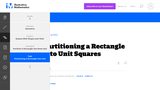Conditional Remix & Share Permitted
CC BY-NC-SA
Rating
0.0 stars

This is a task from the Illustrative Mathematics website that is one part of a complete illustration of the standard to which it is aligned. Each task has at least one solution and some commentary that addresses important aspects of the task and its potential use.

Subject:
Geometry
Material Type:
Activity/Lab
Provider:
Illustrative Mathematics
Provider Set:
Illustrative Mathematics
10/10/2017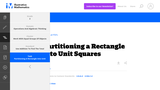Conditional Remix & Share Permitted
CC BY-NC-SA
Rating
0.0 stars

This is a task from the Illustrative Mathematics website that is one part of a complete illustration of the standard to which it is aligned. Each task has at least one solution and some commentary that addresses important aspects of the task and its potential use.

Subject:
Geometry
Material Type:
Activity/Lab
Provider:
Illustrative Mathematics
Provider Set:
Illustrative Mathematics
10/10/2017Conditional Remix & Share Permitted
CC BY-NC-SA
Rating
0.0 stars

This is a task from the Illustrative Mathematics website that is one part of a complete illustration of the standard to which it is aligned. Each task has at least one solution and some commentary that addresses important asects of the task and its potential use. Here are the first few lines of the commentary for this task: * Color the inside of all the triangles blue. * Color the inside of all the quadrilaterals red. * Color the inside of all the pentagons orange. * Color...

Subject:
Mathematics
Material Type:
Activity/Lab
Provider:
Illustrative Mathematics
Provider Set:
Illustrative Mathematics
Author:
Illustrative Mathematics
05/23/2013Conditional Remix & Share Permitted
CC BY-NC-SA
Rating
0.0 stars

This is a task from the Illustrative Mathematics website that is one part of a complete illustration of the standard to which it is aligned. Each task has at least one solution and some commentary that addresses important asects of the task and its potential use. Here are the first few lines of the commentary for this task: Materials * Alexander, Who Used to be Rich Last Sunday by Judith Viorst * Plastic coins * Labels for items Alexander spent his money on (attached) * Pa...

Subject:
Mathematics
Material Type:
Activity/Lab
Provider:
Illustrative Mathematics
Provider Set:
Illustrative Mathematics
Author:
Illustrative Mathematics
06/23/2013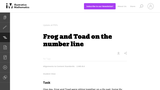Conditional Remix & Share Permitted
CC BY-NC-SA
Rating
0.0 stars

This is a task from the Illustrative Mathematics website that is one part of a complete illustration of the standard to which it is aligned. Each task has at least one solution and some commentary that addresses important asects of the task and its potential use. Here are the first few lines of the commentary for this task: One day, Frog and Toad were sitting together on a lily pad. Some lily pads were in a line across the pond. In the morning, Frog hopped three lily pads ...

Subject:
Mathematics
Material Type:
Activity/Lab
Provider:
Illustrative Mathematics
Provider Set:
Illustrative Mathematics
Author:
Illustrative Mathematics
03/16/2013Conditional Remix & Share Permitted
CC BY-NC-SA
Rating
0.0 stars

This is a task from the Illustrative Mathematics website that is one part of a complete illustration of the standard to which it is aligned. Each task has at least one solution and some commentary that addresses important asects of the task and its potential use. Here are the first few lines of the commentary for this task: Materials * How Big is a Foot? by Rolf Myller * Masking tape * Enough 12-inch (one foot) rulers for every student or pair of students * One yardstick *...

Subject:
Mathematics
Material Type:
Activity/Lab
Provider:
Illustrative Mathematics
Provider Set:
Illustrative Mathematics
Author:
Illustrative Mathematics
05/22/2013Conditional Remix & Share Permitted
CC BY-NC-SA
Rating
0.0 stars

This is a task from the Illustrative Mathematics website that is one part of a complete illustration of the standard to which it is aligned. Each task has at least one solution and some commentary that addresses important asects of the task and its potential use. Here are the first few lines of the commentary for this task: Materials * Sets of 3-6 "analog clock cards," enough for each student * Sets of 3-6 "digital clock cards," enough for each student * Paper and pencil A...

Subject:
Mathematics
Material Type:
Activity/Lab
Provider:
Illustrative Mathematics
Provider Set:
Illustrative Mathematics
Author:
Illustrative Mathematics
05/22/2013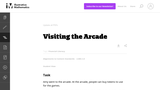Conditional Remix & Share Permitted
CC BY-NC-SA
Rating
0.0 stars

This is a task from the Illustrative Mathematics website that is one part of a complete illustration of the standard to which it is aligned. Each task has at least one solution and some commentary that addresses important asects of the task and its potential use. Here are the first few lines of the commentary for this task: Amy went to the arcade. At the arcade, people can buy tokens to use for the games. Amy paid \5 to get some tokens. Show two different ways she could h... Subject: Mathematics Material Type: Activity/Lab Provider: Illustrative Mathematics Provider Set: Illustrative Mathematics Author: Illustrative Mathematics Date Added: 03/24/2013Conditional Remix & Share Permitted CC BY-NC-SA Rating 0.0 stars This is a task from the Illustrative Mathematics website that is one part of a complete illustration of the standard to which it is aligned. Each task has at least one solution and some commentary that addresses important asects of the task and its potential use. Here are the first few lines of the commentary for this task: 127 is a number. Write it as a sum of 100's, 10's, and 1's. Write its name in words. Draw a picture to represent the number. Locate it on the number li... Subject: Mathematics Material Type: Activity/Lab Provider: Illustrative Mathematics Provider Set: Illustrative Mathematics Author: Illustrative Mathematics Date Added: 05/22/2013Conditional Remix & Share Permitted CC BY-NC-SA Rating 0.0 stars This is a task from the Illustrative Mathematics website that is one part of a complete illustration of the standard to which it is aligned. Each task has at least one solution and some commentary that addresses important asects of the task and its potential use. Here are the first few lines of the commentary for this task: Materials For each student: * A large index card * A pencil Action Part 1 The teacher will put up the following addition problem: \begin{align} 24 &\ \... Subject: Mathematics Material Type: Activity/Lab Provider: Illustrative Mathematics Provider Set: Illustrative Mathematics Author: Illustrative Mathematics Date Added: 03/25/2014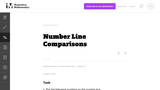Conditional Remix & Share Permitted CC BY-NC-SA Rating 0.0 stars This is a task from the Illustrative Mathematics website that is one part of a complete illustration of the standard to which it is aligned. Each task has at least one solution and some commentary that addresses important asects of the task and its potential use. Here are the first few lines of the commentary for this task: Plot the following numbers on the number line. 456 \ \ 983\ \ 938 \ \ 425 \ \ 220 \ \ 202\ \ 799 Choose eight pairs of numbers from those you plotted o... Subject: Mathematics Material Type: Activity/Lab Provider: Illustrative Mathematics Provider Set: Illustrative Mathematics Author: Illustrative Mathematics Date Added: 04/04/2014Conditional Remix & Share Permitted CC BY-NC-SA Rating 0.0 stars This is a task from the Illustrative Mathematics website that is one part of a complete illustration of the standard to which it is aligned. Each task has at least one solution and some commentary that addresses important asects of the task and its potential use. Here are the first few lines of the commentary for this task: Materials * Whiteboard or chart paper and markers * Empty number line or magnetic cubes lined up on the whiteboard, alternating colors every 5 (see sol... Subject: Mathematics Material Type: Activity/Lab Provider: Illustrative Mathematics Provider Set: Illustrative Mathematics Author: Illustrative Mathematics Date Added: 06/23/2013Conditional Remix & Share Permitted CC BY-NC-SA Rating 0.0 stars This is a task from the Illustrative Mathematics website that is one part of a complete illustration of the standard to which it is aligned. Each task has at least one solution and some commentary that addresses important asects of the task and its potential use. Here are the first few lines of the commentary for this task: Materials * Number cards labeled 1-10 (attached as a PDF) Actions * Begin by playing the game as a whole class to demonstrate the rules and for student... Subject: Mathematics Material Type: Activity/Lab Provider: Illustrative Mathematics Provider Set: Illustrative Mathematics Author: Illustrative Mathematics Date Added: 06/23/2013Conditional Remix & Share Permitted CC BY-NC-SA Rating 0.0 stars This is a task from the Illustrative Mathematics website that is one part of a complete illustration of the standard to which it is aligned. Each task has at least one solution and some commentary that addresses important asects of the task and its potential use. Here are the first few lines of the commentary for this task: Louis wants to give \15 to help kids who need school supplies. He also wants to buy a pair of shoes for \39. How much money will he have to save for ... Subject: Mathematics Material Type: Activity/Lab Provider: Illustrative Mathematics Provider Set: Illustrative Mathematics Author: Illustrative Mathematics Date Added: 01/02/2013 Remix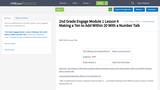Conditional Remix & Share Permitted CC BY-NC Rating 0.0 stars This is a modified lesson 4 module 1 grade 2 from EngageNY, make a ten to add within 20. Subject: Education Mathematics Material Type: Lesson Author: Lisa Jarvensivu Date Added: 08/21/2019 RemixConditional Remix & Share Permitted CC BY-NC-SA Rating 0.0 stars Students will create a 3D model comprised of 3 different shapes. This will be done with Play-doh or in Tinkercad. They will then find the volume and surface area of the composite 3D shape. Subject: Mathematics Material Type: Lesson Author: Lynn Aprill Nicholas Schaumberg Date Added: 09/04/2019 RemixConditional Remix & Share Permitted CC BY-NC-SA Rating 0.0 stars Students will create a 3D model comprised of 3 different shapes. This will be done with Play-doh or in Tinkercad. They will then find the volume and surface area of the composite 3D shape. Subject: Mathematics Material Type: Lesson Author: Lynn Aprill Nicholas Schaumberg Date Added: 11/19/2019Conditional Remix & Share Permitted CC BY-NC-SA Rating 0.0 stars This is a task from the Illustrative Mathematics website that is one part of a complete illustration of the standard to which it is aligned. Each task has at least one solution and some commentary that addresses important asects of the task and its potential use. Here are the first few lines of the commentary for this task: A small square is a square unit. What is the area of this rectangle? Explain. What fraction of the area of each rectangle is shaded blue? Name the frac... Subject: Mathematics Material Type: Activity/Lab Provider: Illustrative Mathematics Provider Set: Illustrative Mathematics Author: Illustrative Mathematics Date Added: 05/16/2013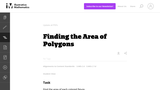Conditional Remix & Share Permitted CC BY-NC-SA Rating 0.0 stars This is a task from the Illustrative Mathematics website that is one part of a complete illustration of the standard to which it is aligned. Each task has at least one solution and some commentary that addresses important asects of the task and its potential use. Here are the first few lines of the commentary for this task: Find the area of each colored figure. Each grid square is 1 inch long.... Subject: Mathematics Material Type: Activity/Lab Provider: Illustrative Mathematics Provider Set: Illustrative Mathematics Author: Illustrative Mathematics Date Added: 05/27/2013Conditional Remix & Share Permitted CC BY-NC-SA Rating 0.0 stars This is a task from the Illustrative Mathematics website that is one part of a complete illustration of the standard to which it is aligned. Each task has at least one solution and some commentary that addresses important aspects of the task and its potential use. Subject: Measurement and Data Material Type: Activity/Lab Provider: Illustrative Mathematics Provider Set: Illustrative Mathematics Date Added: 10/10/2017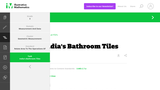Conditional Remix & Share Permitted CC BY-NC-SA Rating 0.0 stars This is a task from the Illustrative Mathematics website that is one part of a complete illustration of the standard to which it is aligned. Each task has at least one solution and some commentary that addresses important aspects of the task and its potential use. Subject: Measurement and Data Material Type: Activity/Lab Provider: Illustrative Mathematics Provider Set: Illustrative Mathematics Date Added: 10/10/2017Conditional Remix & Share Permitted CC BY-NC-SA Rating 0.0 stars This is a task from the Illustrative Mathematics website that is one part of a complete illustration of the standard to which it is aligned. Each task has at least one solution and some commentary that addresses important aspects of the task and its potential use. Subject: Measurement and Data Material Type: Activity/Lab Provider: Illustrative Mathematics Provider Set: Illustrative Mathematics Date Added: 10/10/2017Conditional Remix & Share Permitted CC BY-NC-SA Rating 0.0 stars This is a task from the Illustrative Mathematics website that is one part of a complete illustration of the standard to which it is aligned. Each task has at least one solution and some commentary that addresses important asects of the task and its potential use. Here are the first few lines of the commentary for this task: Draw a purple pentagon Draw a blue shape with 3 line segments that is not a triangle. Draw an orange shape with 4 line segments that is not a quadrilat... Subject: Mathematics Material Type: Activity/Lab Provider: Illustrative Mathematics Provider Set: Illustrative Mathematics Author: Illustrative Mathematics Date Added: 05/27/2013Conditional Remix & Share Permitted CC BY-NC-SA Rating 0.0 stars This is a task from the Illustrative Mathematics website that is one part of a complete illustration of the standard to which it is aligned. Each task has at least one solution and some commentary that addresses important asects of the task and its potential use. Here are the first few lines of the commentary for this task: Plot the following numbers on the number line: 80 328 791 1. Round each number to the nearest 100. How can you see this on the number line? 2. Round ea... Subject: Mathematics Material Type: Activity/Lab Provider: Illustrative Mathematics Provider Set: Illustrative Mathematics Author: Illustrative Mathematics Date Added: 02/18/2014Conditional Remix & Share Permitted CC BY-NC-SA Rating 0.0 stars This is a task from the Illustrative Mathematics website that is one part of a complete illustration of the standard to which it is aligned. Each task has at least one solution and some commentary that addresses important asects of the task and its potential use. Here are the first few lines of the commentary for this task: There are 6 tables in Mrs. Potter's art classroom. There are 4 students sitting at each table. Each student has a box of 10 colored pencils. (A) How ma... Subject: Mathematics Material Type: Activity/Lab Provider: Illustrative Mathematics Provider Set: Illustrative Mathematics Author: Illustrative Mathematics Date Added: 05/17/2013Conditional Remix & Share Permitted CC BY-NC-SA Rating 0.0 stars This is a task from the Illustrative Mathematics website that is one part of a complete illustration of the standard to which it is aligned. Each task has at least one solution and some commentary that addresses important asects of the task and its potential use. Here are the first few lines of the commentary for this task: Plot 8, 32, and 79 on the number line. 1. Round each number to the nearest 10. How can you see this on the number line? 2. Round each number to the nea... Subject: Mathematics Material Type: Activity/Lab Provider: Illustrative Mathematics Provider Set: Illustrative Mathematics Author: Illustrative Mathematics Date Added: 02/18/2014Conditional Remix & Share Permitted CC BY-NC-SA Rating 0.0 stars This is a task from the Illustrative Mathematics website that is one part of a complete illustration of the standard to which it is aligned. Each task has at least one solution and some commentary that addresses important asects of the task and its potential use. Here are the first few lines of the commentary for this task: Choose each statement that is true.\frac34$is greater than$\frac54$.$\frac54$is greater than$\frac34$.$\frac34 \gt \frac54$.$\frac34 \lt \frac...

Subject:
Mathematics
Material Type:
Activity/Lab
Provider:
Illustrative Mathematics
Provider Set:
Illustrative Mathematics
Author:
Illustrative Mathematics
03/18/2013Conditional Remix & Share Permitted
CC BY-NC-SA
Rating
0.0 stars

This is a task from the Illustrative Mathematics website that is one part of a complete illustration of the standard to which it is aligned. Each task has at least one solution and some commentary that addresses important asects of the task and its potential use. Here are the first few lines of the commentary for this task: Choose each statement that is true. $\frac98$ is greater than $\frac{9}{4}$. $\frac{9}{4}$ is greater than $\frac98$. $\frac98 \gt \frac{9}{4}$. \frac... Subject: Mathematics Material Type: Activity/Lab Provider: Illustrative Mathematics Provider Set: Illustrative Mathematics Author: Illustrative Mathematics Date Added: 03/18/2013Conditional Remix & Share Permitted CC BY-NC-SA Rating 0.0 stars This is a task from the Illustrative Mathematics website that is one part of a complete illustration of the standard to which it is aligned. Each task has at least one solution and some commentary that addresses important asects of the task and its potential use. Here are the first few lines of the commentary for this task: The number line below shows two numbers, 0 and 1. Where is\frac14on this number line? http://www.youtube.com/watch?v=HJ0LDgxVGfU... Subject: Mathematics Material Type: Activity/Lab Provider: Illustrative Mathematics Provider Set: Illustrative Mathematics Author: Illustrative Mathematics Date Added: 03/18/2013Conditional Remix & Share Permitted CC BY-NC-SA Rating 0.0 stars This is a task from the Illustrative Mathematics website that is one part of a complete illustration of the standard to which it is aligned. Each task has at least one solution and some commentary that addresses important asects of the task and its potential use. Here are the first few lines of the commentary for this task: The number line below shows two numbers, 0 and\frac53. Where is 1 on this number line? http://www.youtube.com/watch?v=wAXRjMUgiu4... Subject: Mathematics Material Type: Activity/Lab Provider: Illustrative Mathematics Provider Set: Illustrative Mathematics Author: Illustrative Mathematics Date Added: 03/18/2013Conditional Remix & Share Permitted CC BY-NC-SA Rating 0.0 stars This is a task from the Illustrative Mathematics website that is one part of a complete illustration of the standard to which it is aligned. Each task has at least one solution and some commentary that addresses important asects of the task and its potential use. Here are the first few lines of the commentary for this task: The number line below shows two numbers, 0 and 1. Where is\frac74on this number line? http://www.youtube.com/watch?v=TEzH_PbHZIw... Subject: Mathematics Material Type: Activity/Lab Provider: Illustrative Mathematics Provider Set: Illustrative Mathematics Author: Illustrative Mathematics Date Added: 03/18/2013Conditional Remix & Share Permitted CC BY-NC-SA Rating 0.0 stars This is a task from the Illustrative Mathematics website that is one part of a complete illustration of the standard to which it is aligned. Each task has at least one solution and some commentary that addresses important asects of the task and its potential use. Here are the first few lines of the commentary for this task: Who correctly compares the numbers 2/3 and 2/5? Ben said that 2/3 is greater than 2/5. Lee said that 2/3 is equal to 2/5. Mia said that 2/3 is less tha... Subject: Mathematics Material Type: Activity/Lab Provider: Illustrative Mathematics Provider Set: Illustrative Mathematics Author: Illustrative Mathematics Date Added: 03/18/2013Conditional Remix & Share Permitted CC BY-NC-SA Rating 0.0 stars This is a task from the Illustrative Mathematics website that is one part of a complete illustration of the standard to which it is aligned. Each task has at least one solution and some commentary that addresses important asects of the task and its potential use. Here are the first few lines of the commentary for this task: Alec and Felix are brothers who go to different schools. The school day is just as long at Felix' school as at Alec's school. At Felix' school, there a... Subject: Mathematics Material Type: Activity/Lab Provider: Illustrative Mathematics Provider Set: Illustrative Mathematics Author: Illustrative Mathematics Date Added: 05/17/2013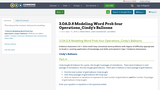Conditional Remix & Share Permitted CC BY-NC-SA Rating 0.0 stars This task provides students with practice modeling. Subject: Education Mathematics Material Type: Activity/Lab Assessment Homework/Assignment Author: Linda Schoenbrodt Lisa Adkins MSDE Admin Date Added: 02/16/2022Conditional Remix & Share Permitted CC BY-NC-SA Rating 0.0 stars This is a task from the Illustrative Mathematics website that is one part of a complete illustration of the standard to which it is aligned. Each task has at least one solution and some commentary that addresses important asects of the task and its potential use. Here are the first few lines of the commentary for this task: Tehya and Kenneth are trying to figure out which number could be placed in the box to make this equation true. Tehya insists that 12 is the only number... Subject: Mathematics Material Type: Activity/Lab Provider: Illustrative Mathematics Provider Set: Illustrative Mathematics Author: Illustrative Mathematics Date Added: 02/18/2014Conditional Remix & Share Permitted CC BY-NC-SA Rating 0.0 stars This is a task from the Illustrative Mathematics website that is one part of a complete illustration of the standard to which it is aligned. Each task has at least one solution and some commentary that addresses important asects of the task and its potential use. Here are the first few lines of the commentary for this task: Suppose there are 4 tanks and 3 fish in each tank. The total number of fish in this situation can be expressed as4 \times 3 = 12. Describe what is m... Subject: Mathematics Material Type: Activity/Lab Provider: Illustrative Mathematics Provider Set: Illustrative Mathematics Author: Illustrative Mathematics Date Added: 08/13/2013Conditional Remix & Share Permitted CC BY-NC-SA Rating 0.0 stars This is a task from the Illustrative Mathematics website that is one part of a complete illustration of the standard to which it is aligned. Each task has at least one solution and some commentary that addresses important asects of the task and its potential use. Here are the first few lines of the commentary for this task: Presley has 18 markers. Her teacher gives her three boxes and asks her to put an equal number of markers in each box. Anthony has 18 markers. His teach... Subject: Mathematics Material Type: Activity/Lab Provider: Illustrative Mathematics Provider Set: Illustrative Mathematics Author: Illustrative Mathematics Date Added: 09/07/2013Conditional Remix & Share Permitted CC BY-NC-SA Rating 0.0 stars This is a task from the Illustrative Mathematics website that is one part of a complete illustration of the standard to which it is aligned. Each task has at least one solution and some commentary that addresses important asects of the task and its potential use. Here are the first few lines of the commentary for this task: Mrs. Moore’s third grade class wants to go on a field trip to the science museum. * The cost of the trip is \245. * The class can earn money by runnin...

Subject:
Mathematics
Material Type:
Activity/Lab
Provider:
Illustrative Mathematics
Provider Set:
Illustrative Mathematics
Author:
Illustrative Mathematics